A major cause of the replication crisis that currently plagues the life sciences is low statistical power. But power is a nebulous concept, and power calculations are rarely used to plan experiments (and still more rarely used sensibly). In this notebook I introduce basic power calculation terminology, and cover how the positive and negative predictive value quantifies how statistical power and significance thresholding influences reproducibility. Stay tuned for the next notebook, where we discuss effect sizes and sample size calculations.

I work in human neuroimaging and psychology. Researchers in these fields are increasingly concerned about reproducibility. Informally, that means we worry that a lot of published claims aren't true. We can formalise this intuition in terms of the positive predictive value (PPV), that is, the proportion of reported statistically significant effects that represent a true effect in the population of effects that the sample came from. Ioannidis (2005) famously argued that if we estimate the PPV of an entire literature, there are possible scenarios where PPV is less than 0.5. That is, most published significant effects in that literature are actually false positives (see also Berger & Sellke, 1987). It's difficult to imagine that cumulative science can happen under such conditions (especially if non-significant results are left in the file drawer rather than reported). What's going wrong?

Poor reproducibility has two basic causes. First, questionable research practices such as the file drawer effect and the garden of forking paths [pdf] (sometimes more pejoratively described as p-hacking), which bias reported effect sizes and inferential statistics. I think most researchers are aware of these issues by now, although the cures (pre-registration, validation samples) have yet to be widely adopted. The second cause of poor reproducibility is less widely understood: low statistical power, and overly-liberal significance thresholding. As Ioannidis (2005) argued, there are plausible conditions under which a statistically significant test is more likely to reflect a null than a true effect, even in the absence of any questionable research practices. This notebook is an attempt to make this more concrete. I will start with a refresher on definitions, but feel free to skip ahead to the code, where we explore expected reproducibility in a few scenarios.

If you want to run the notebook yourself, it is available on my github.

In :
# imports
import matplotlib.pyplot as plt
import matplotlib
font = {'size'   : 12}
matplotlib.rc('font', **font)
import numpy as np
%config InlineBackend.figure_format = 'retina'
from statsmodels.stats import power
ttest_paired = power.TTestPower()


## A quick review of alpha, power, and predictive values¶

### Terminology¶

Here is a little reminder of a classic null hypothesis testing contingency table, with common labels for each cell:

statistical test null true null false
significant $\alpha$ false positive rate, false alarm, type 1 error $1-\beta$ power, sensitivity, hit
not significant $1-\alpha$ specificity, correct rejection $\beta$ false negative rate, miss, type 2 error
• $p$ is the probability of observing a test statistic of this size or greater when the null hypothesis is true
• $\alpha$ is the significance threshold we adopt for $p$ (conventionally 0.05). We reject the null hypothesis when $p < \alpha$. We generally refer to this as the false positive rate, because we expect to report a false positive on $\alpha$ proportion of the tests we calculate when the null hypothesis is true
• $\beta$ is the false negative rate. It represents the proportion of statistical tests that we expect to be non-significant for a given effect size and $\alpha$ when the null hypothesis is false
• $1-\beta$ is the power of a statistical test (conventionally fixed at 0.8 for sample size calculations). It indicates how often an experiment is expected to produce a statistically significant effect for a given effect size when the null hypothesis is false

Notice that the conventional parameters for significance threshold and power ($\alpha=0.05, (1-\beta)=0.8$) represent a particular tradeoff, where we accept a 4 times higher false negative rate relative to the false positive rate. A popular alternative is to calculate sample sizes for 0.95 power ($1-\alpha$), which corresponds to equal control of false positive and false negative rates. This seems a sensible tradeoff if we are using statistical significance thresholds to decide whether a research programme is 'promising', that is, whether to build on a result with further experiments.

What's missing from this analysis is the base rate, that is, the proportion of the tested hypotheses that are true. As we will see next, this prevalence parameter is crucial for fully characterizing the expected properties of our hypothesis-testing approach.

### The positive predictive value (PPV) and prevalence¶

To calculate the PPV of a literature, that is, the proportion of all reported significant tests that are true positives, we need one more thing: effect prevalence, that is, the proportion of tested hypotheses that are true. If $\text{prevalence}=1$, all hypotheses are true, and any non-significant test is actually a false negative. If $\text{prevalence}=0$, all hypotheses are false, and all significant tests are actually false positives. Once we have fixed prevalence, PPV is simply the true positives scaled by the sum of the true and false positives:

$$\text{PPV} = \frac{(1-\beta) \times \text{prevalence}}{(1-\beta) \times \text{prevalence}+\alpha \times (1-\text{prevalence})}$$

On the numerator, recall that $(1-\beta)$ is power, ie the proportion of the true hypotheses we test where we expect to obtain statistical significance. We need to scale this by prevalence to consider how common true hypotheses are. The denominator is the sum of this and the false positive rate $\alpha$ scaled by how often we expect to encounter false hypotheses (the remaining of the cases, so $(1-\text{prevalence})$).

At this point, you might be wondering what a reasonable value for prevalence would be. We don't know! And the file drawer problem makes it difficult to estimate from published results. It might be 0 in parapsychology, and close to 1 for a lot of other areas of psychology (for instance, it's sometimes hard to imagine that stated hypotheses in applied or health psychology could be false). We'll return to this point in the code below.

#### A boring note on terminology¶

In this notebook we refer to the proportion of reported significant tests that are true positives as the literature PPV, following Ioannidis (2005). Subsequent work tended to report the complement, ie the proportion of reported significant tests that are false positives $(1-\text{PPV})$. This quantity goes by various names: false discovery rate (Colquhoun, 2014), false positive rate (Benjamin et al., 2018), false positive risk (Colquhoun, 2019), false report probability (Szucs & Ioannidis, 2017). It's also worth remembering that PPV is referred to as precision in machine learning contexts. Some of these terms are worse than others - I like literature PPV because it doesn't invite confusion with other statistical concepts.

While we're muddying the waters, let's also note that many investigators prefer to express prevalence in terms of odds of the alternate hypothesis being true, typically denoted $R$ (e.g., Ioannidis, 2005). In this formulation, $R = \text{prevalence} / (1-\text{prevalence})$ and we can express PPV as

$$\text{PPV} = \frac{(1-\beta) \times R}{(1-\beta) \times R + \alpha}$$

This formula is undeniably tidier but I am not Bayesian enough to find odds less confusing than proportions, so we will stick with the latter here.

### The negative predictive value (NPV)¶

We can estimate a literature's negative predictive value through a very similar calculation as PPV, but applied to the lower row of the null hypothesis testing contingency table above. That is, when we fail to reject the null hypothesis, how often does this reflect a true null effect?

$$\text{NPV} = \frac{(1-\alpha) \times (1-\text{prevalence})}{(1-\alpha) \times (1-\text{prevalence}) + \beta \times \text{prevalence}}$$

On the numerator we need the specificity of the test $(1-\alpha)$ scaled by the proportion of true nulls $(1-\text{prevalence})$. The denominator is the sum of this and the false negative rate scaled by the proportion of true hypotheses ($\beta \times \text{prevalence}$).

It's worth flagging up already at this point that thinking about NPV for a literature really makes explicit the intellectual contortions of the conventional Neyman-Pearson null hypothesis significance testing framework: these PPV and NPV calculations only strictly hold for a funny dichotomous world where effects are exactly null, or not null and of the size that we power our analysis to detect. Which brings us to the next point...

### I don't know this is starting to sound a bit Bayesian¶

Why yes! In Bayesian terms, PPV is the posterior probability of a true hypothesis given a significant test, and $1-\text{NPV}$ is the posterior probability of a true hypothesis given a non-significant effect (Johnson et al, 2001 - thanks to Will Penny for bringing this connection to my attention). It turns out that we can't avoid prior belief (ie, the prevalence parameter) if we want to estimate PPV and NPV. But the Bayesians in the back of the room would probably object to the entire premise of discretizing evidence into arbitrary 'significant' and 'non-significant' categories, when we could be characterizing the entire posterior distribution instead... We may return to Bayesian alternatives to power analysis in a future post.

## Exploring positive and negative predictive values in different scenarios¶

The title claim in Ioannidis (2005) that most published claims are false holds specifically when $\text{prevalence}<0.5$, power is low, and statistical significance is assessed at the conventional $\alpha=0.05$ level. Whether that's a reasonable description of the state of your research area is for you to judge. To make this more concrete, let's explore the PPV and NPV consequences of different power and prevalence combinations.

In :
# in python, PPV and NPV can be calculated as
# notice that we use power (1-beta) as input instead of beta here
ppv = lambda power, alpha, prevalence: (power * prevalence) / (
power * prevalence + alpha * (1 - prevalence)
)
# or odds formulation if you prefer
ppv_odds = lambda power, alpha, R: (power * R) / (power * R + alpha)
npv = lambda power, alpha, prevalence: ((1 - alpha) * (1 - prevalence)) / (
(1 - alpha) * (1 - prevalence) + (1 - power) * prevalence
)

# we can also visualise the scenario with a pie chart like so:
def power_pie(power, alpha, prevalence):
# we can either 'hit' or 'miss' true positives
hits = power * prevalence
misses = (1 - power) * prevalence
# and for true positives, we can have false positives and correct rejections
false_positives = alpha * (1 - prevalence)
correct_rejections = (1 - alpha) * (1 - prevalence)
ax = plt.gca()
ax.pie(
(hits, misses, false_positives, correct_rejections),
labels=(f"H1, p<{alpha}", f"H1, p>{alpha}", f"H0, p<{alpha}", f"H0, p>{alpha}"),
colors=("darkslateblue", "slateblue", "darkgoldenrod", "goldenrod"),
)
return


### Scenario 1¶

Let's say we are doing discovery science (only 25% of hypotheses are true) and let's further assume we are good scientists, who power studies to the conventional 80% level:

In :
# this idiom is useful to avoid typos when the same args are passed to multiple functions
good_args = dict(power=0.8, alpha=0.05, prevalence=0.25)
good = ppv(**good_args)
print(
f"well-powered discovery scientists...\nare right {good*100:.3}% "
"of the times they declare significance"
)
good_neg = npv(**good_args)
print(
f"and are right {good_neg*100:.3}% "
"of the times they fail to declare significance"
)
fig, ax = plt.subplots(1, 1, figsize=[3, 3],)
power_pie(**good_args)

well-powered discovery scientists...
are right 84.2% of the times they declare significance
and are right 93.4% of the times they fail to declare significance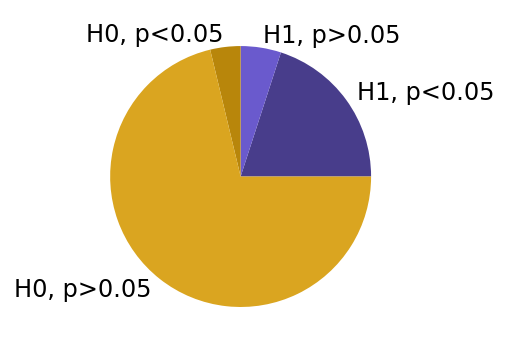Not too bad, right? If this seems like your field you might be sleeping quite soundly at night.

### Scenario 2¶

Now suppose we work in the same field, but we are 'bad' scientists who haven't powered our studies appropriately. Or perhaps we are the very same scientists, but it turns out we were wildly off base on the true effect magnitude.

In :
bad_args = dict(power=0.2, alpha=0.05, prevalence=0.25)
print(
f"underpowered discovery scientists...\nare right {bad*100:.3}% "
"of the times they declare significance"
)
print(
"of the times they fail to declare significance"
)
fig, ax = plt.subplots(1, 1, figsize=[3, 3])

underpowered discovery scientists...
are right 57.1% of the times they declare significance
and are right 78.1% of the times they fail to declare significance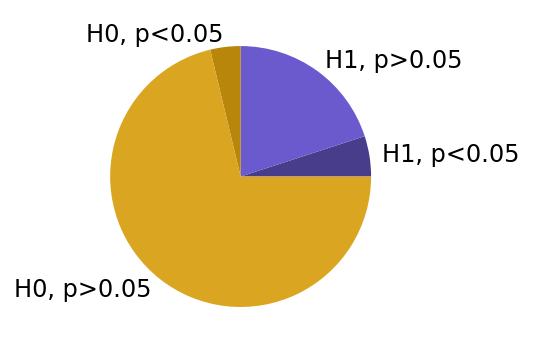We are now perilously close to that $\text{PPV}<0.5$ regime that led Ioannidis (2005) to claim that most published findings are false. Notice that NPV remains decent because the prevalence of true hypotheses is low (you can't miss something that isn't there to detect).

### Scenario 3¶

Let's next imagine we are good scientists with adequate power, but we work in a field where almost every hypothesis is false (let's call it social priming). For this, we will set the prevalence of true hypotheses lower:

In :
misguided_args = dict(power=0.8, alpha=0.05, prevalence=0.05)
misguided = ppv(**misguided_args)
print(
f"well-powered but misguided scientists...\nare right {misguided*100:.3}% "
"of the times they declare significance"
)
misguided_neg = npv(**misguided_args)
print(
f"and are right {misguided_neg*100:.3}% "
"of the times they fail to declare significance"
)
fig, ax = plt.subplots(1, 1, figsize=[3, 3])
power_pie(**misguided_args)

well-powered but misguided scientists...
are right 45.7% of the times they declare significance
and are right 98.9% of the times they fail to declare significance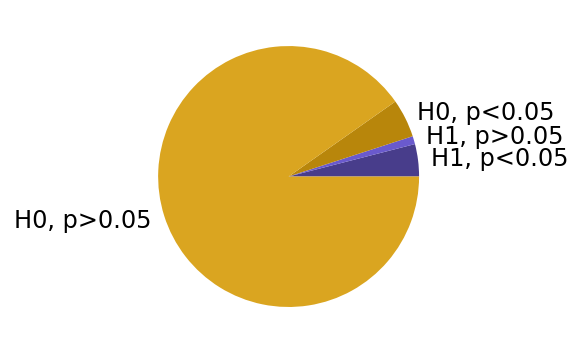Even with adequate power, chasing sufficiently rare effects results in low PPV. Or put differently, powering studies at the conventional 0.8 level is only going to produce trustworthy positive effects if your experiment wasn't a complete shot in the dark in the first place (note again how the Bayesian reasoning creeps into our frequentist tests!).

### Scenario 4¶

Finally, let's suppose we are underpowered, but we work in a field where most of the tested hypotheses are likely to be true anyway (let's call it applied psychology). For this, we set power low and prevalence high:

In :
obvious_arg = dict(power=0.2, alpha=0.05, prevalence=0.7)
obvious = ppv(**obvious_arg)
print(
f"underpowered scientists of the obvious...\nare right {obvious*100:.3}% "
"of the times they declare significance"
)
obvious_neg = npv(**obvious_arg)
print(
f"and are right {obvious_neg*100:.3}% "
"of the times they fail to declare significance"
)
fig, ax = plt.subplots(1, 1, figsize=[3, 3])
power_pie(**obvious_arg)

underpowered scientists of the obvious...
are right 90.3% of the times they declare significance
and are right 33.7% of the times they fail to declare significance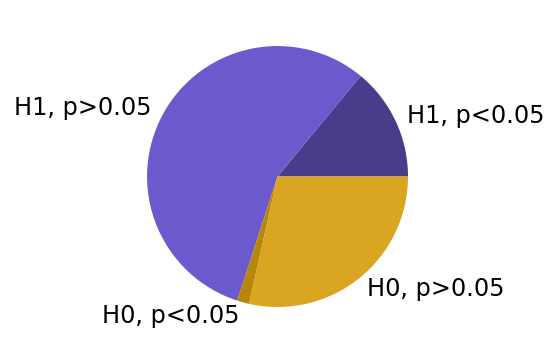Notice that low power does not necessarily produce low PPV, if you're mostly making 'safe' predictions. But the price you pay for low power in this scenario is poor NPV - there are lot of other hypotheses that are also true, but you can't detect them.

Taken together, these scenarios illustrate that:

• Low statistical power affects both PPV and NPV. It's a common misconception that we don't have to worry about power as long as we have achieved statistical significance (for a somewhat tongue-in-cheek example of this type of reasoning, see Friston, 2012). But as we have seen, low power means lower NPV and PPV. If we suspect that power is low in our field, we should be less confident in both significant and non-significant results.
• If we work in a field where the prevalence of true effects is low, high statistical power won't save us from low PPV. Even if we detect true effects resonably well when they are there, PPV ends up dominated by the false positives. We should be skeptical of claims from fields that pursue 'high-risk' experiments, even if a lot of data is presented (ie, power is likely high for the putative effect). The Bem ESP debacle can be considered an example of this scenario.
• If we work in a field where null hypothesis tests are reported as 'sanity checks' for effects that everyone already basically believes in (rather than to claim a genuine new discovery), PPV can remain quite high even if power is low. But the price we pay is low NPV. A researcher in such a field might have the frustrating experience that experiments that really 'should' work often won't.

### The relationship between prevalence, power, and significance thresholding¶

One theme that emerges from these scenarios is that if we plan our research according to currently-conventional cutoffs for significance and power ($\alpha=0.05, (1-\beta)=0.8$), we can only expect to observe high PPV and NPV within a fairly restricted range of effect prevalence rates. There is a sort of prevalence 'sweet spot' for which these standard parameters work well.

But what if statistical power in your field is actually more like 20%, as has been claimed for neuroscience research (Button et al, 2013)? Or what if we power to a more ambitious 95%, as I argued for above? Let's explore this more systematically.

In :
# control variable for the following plots
prevalence_rates = np.linspace(0, 1, 100)
sweet = 0.8
effect = 0.5

def panel_prevalence(this_ax, this_power, this_alpha):
this_ppv = ppv(power=this_power, alpha=this_alpha, prevalence=prevalence_rates)
this_npv = npv(power=this_power, alpha=this_alpha, prevalence=prevalence_rates)
# shade range where both ppv and npv are over sweet threshold
this_patch = this_ax.fill_between(
prevalence_rates,
0,
1,
where=(this_ppv > sweet) & (this_npv > sweet),
color=(0.8, 0.9, 0.8),
)
this_ax.set_xlabel(f"prevalence\n(power={this_power})")
# insert example sample size N to make this a bit more concrete
example_n = np.ceil(
ttest_paired.solve_power(
effect_size=effect,
alpha=this_alpha,
power=this_power,
alternative="two-sided",
)
)
th = this_ax.text(
0,
1,
f"$N$={example_n:.0f}",
verticalalignment="bottom",
horizontalalignment="left",
transform=this_ax.transAxes,
)
return this_ax.plot(
prevalence_rates, np.hstack((this_npv[:, None], this_ppv[:, None])), linewidth=3
)

# first set of panels at standard alpha - 0.05
this_alpha = 0.05
fig, ax = plt.subplots(1, 3, sharex=True, sharey=True, figsize=(8, 2))
# ylabel in first panel
ax.set_ylabel(f"rate\n($\\alpha$={this_alpha})")
for this_power, this_ax in zip((0.2, 0.8, 0.95), ax):
this_ph = panel_prevalence(this_ax, this_power, this_alpha)
# legend in final panel
lh = this_ax.legend(this_ph, ("NPV", "PPV"))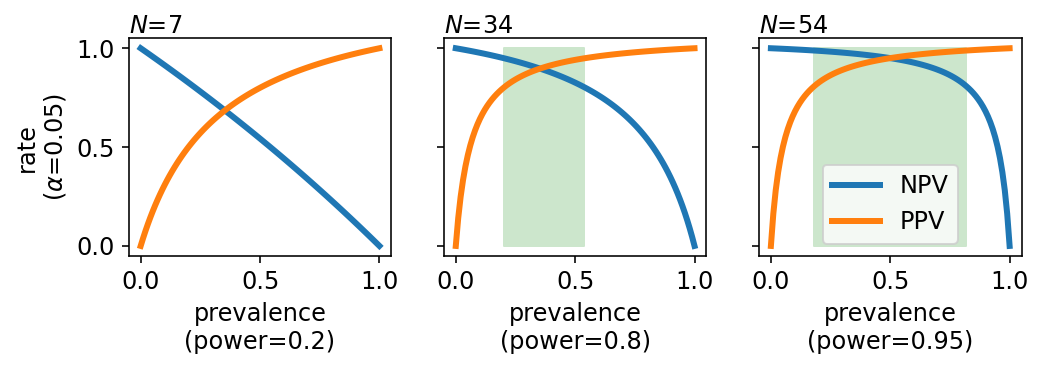These plots illustrate PPV and NPV as a function of effect prevalence. The panels show different power parameters. To make this a bit more concrete, I have included an example sample size calculation $N$ for a moderate within-subjects mean difference (paired $T$, two-tailed, $d=0.5$) above each panel. Let's unpack what we see:

• That prevalence 'sweet spot' we saw a hint of in the examples above is the shaded green range where both PPV (blue) and NPV (yellow) curves are over some threshold (here I used 0.8). Assuming that we care equally about PPV and NPV, this is the prevalence range over which the analysis achieves a criterion level. Equal weights on positive and negative predictive values seems reasonable for discovery science, but if our priorities were different we could of course calculate a sweet spot for some other weighting.
• If power is low (0.2, leftmost panel), there is no prevalence rate for which our analysis achieves criterion-level PPV and NPV. Depending on the effect prevalence rate we can have high PPV or high NPV, but not both. For discovery science I think lower effect prevalence rates are more realistic, which means we are in a low PPV regime. These are the conditions that formed the basis of Ioannidis' (2005) argument that most claims are false.
• Increasing power to adequate levels (0.8, middle panel) means we achieve criterion-level PPV and NPV in a fairly narrow 0.25-0.5 prevalence range.
• If we increase power further to $1-\alpha$ (0.95, right panel), we mainly extend the criterion-level range rightward, to high prevalence rates. The NPV curve gets closer to the upper right corner of the plot with higher power, but the PPV curve doesn't change much.

So far, I think the situation is a little disappointing. Even as we increase power to high levels, we don't substantially increase our ability to reliably detect rare effects, because PPV falls off precipitously as prevalence goes below 0.25. We would have power our studies to very high levels indeed to achieve reasonable PPV when effect prevalence is low.

However, other investigators have proposed adopting a stricter $\alpha=0.005$ criterion for statistical significance in discovery science (Benjamin et al, 2018). How would that change things?

In :
this_alpha = 0.005
fig, ax = plt.subplots(1, 3, sharex=True, sharey=True, figsize=(8, 2))
ax.set_ylabel(f"rate\n($\\alpha$={this_alpha})")
for this_power, this_ax in zip((0.2, 0.8, 0.95), ax):
this_ph = panel_prevalence(this_ax, this_power, this_alpha)
lh = this_ax.legend(this_ph, ("NPV", "PPV"))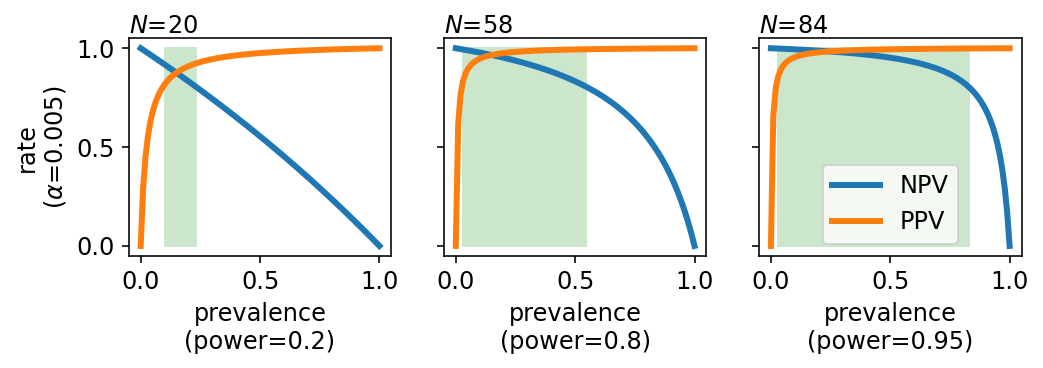Setting a more stringent $\alpha$ (0.05 to 0.005) shifts the effect prevalence sweet spot to lower rates. A conventionally-powered analysis (0.8) now achieves criterion-level PPV and NPV for prevalence rates ranging from about 0.1 to 0.5. This seems desirable if we suspect that effect prevalence is indeed low in our research area (Benjamin et al, 2018 discuss evidence for a rate of 0.09 in psychology).

It is perhaps unrealistic to think that Benjamin et al's (2018) re-defined significance threshold would result in larger samples being collected, since sample sizes are mostly set according to heuristics ($N=20$ is particularly unshakeable in my field), rather than formal power analysis. If we adopt a stricter $\alpha$ without increasing sample sizes our analysis necessarily has reduced statistical power. To appreciate the consequences of lower power in terms of PPV and NPV, we can compare two cases above that have quite similar sample size requirements: adequate power (0.8) at $\alpha=0.005$ (middle panel above) and high power (0.95) at $\alpha=0.05$ (right panel in the previous figure). We can see that the effect of adopting a stricter $\alpha$ by itself is still to shift the effect prevalence sweet spot leftward. The loss in statistical power results in lower NPV, but this effect is relatively subtle at low prevalence rates (since NPV is dominated by the many true negatives in this range).

## Conclusions¶

I hope these calculations clarify how power and $\alpha$ influences expected reproducibility. I'm going to close on a few take-home messages.

• Low power means low trust in reported non-significant and significant tests. It is not the case that we only have to worry about power when we fail to reject the null hypothesis.
• We can't reason about the expected reproducibility of a field (for instance, by calculating PPV and NPV) without considering the effect prevalence rate. This need to express what amounts to prior beliefs can be uncomfortable for frequentists, but I hope you'll agree it's worthwhile.
• It's dangerous to focus narrowly on literature PPV. Cumulative science with null hypothesis significance testing probably requires high NPV as well.
• Unless your research has exceptional statistical power, you will not achieve high PPV for rare effects when the conventional $\alpha=0.05$ significance threshold is used. If we suspect prevalence rates are low, the solution is generally to adopt a stricter criterion for statistical significance.
• Finally, let's remember that these calculations represent the best-case scenario: No questionable research practices that bias the p values, and enough of a handle on effect size to power studies accurately.

In the next notebook, we will turn to the more practical issue of sample size calculations. We will see that this requires an estimate of standardized effect size (e.g., cohen's $d$), and that obtaining an unbiased, low-variance estimate of this from previous studies is hard. Stay tuned for that.